$$\newcommand{\id}{\mathrm{id}}$$ $$\newcommand{\Span}{\mathrm{span}}$$ $$\newcommand{\kernel}{\mathrm{null}\,}$$ $$\newcommand{\range}{\mathrm{range}\,}$$ $$\newcommand{\RealPart}{\mathrm{Re}}$$ $$\newcommand{\ImaginaryPart}{\mathrm{Im}}$$ $$\newcommand{\Argument}{\mathrm{Arg}}$$ $$\newcommand{\norm}{\| #1 \|}$$ $$\newcommand{\inner}{\langle #1, #2 \rangle}$$ $$\newcommand{\Span}{\mathrm{span}}$$

# 4.5: Use the Slope–Intercept Form of an Equation of a Line

•• OpenStax
• OpenStax
$$\newcommand{\vecs}{\overset { \rightharpoonup} {\mathbf{#1}} }$$ $$\newcommand{\vecd}{\overset{-\!-\!\rightharpoonup}{\vphantom{a}\smash {#1}}}$$$$\newcommand{\id}{\mathrm{id}}$$ $$\newcommand{\Span}{\mathrm{span}}$$ $$\newcommand{\kernel}{\mathrm{null}\,}$$ $$\newcommand{\range}{\mathrm{range}\,}$$ $$\newcommand{\RealPart}{\mathrm{Re}}$$ $$\newcommand{\ImaginaryPart}{\mathrm{Im}}$$ $$\newcommand{\Argument}{\mathrm{Arg}}$$ $$\newcommand{\norm}{\| #1 \|}$$ $$\newcommand{\inner}{\langle #1, #2 \rangle}$$ $$\newcommand{\Span}{\mathrm{span}}$$ $$\newcommand{\id}{\mathrm{id}}$$ $$\newcommand{\Span}{\mathrm{span}}$$ $$\newcommand{\kernel}{\mathrm{null}\,}$$ $$\newcommand{\range}{\mathrm{range}\,}$$ $$\newcommand{\RealPart}{\mathrm{Re}}$$ $$\newcommand{\ImaginaryPart}{\mathrm{Im}}$$ $$\newcommand{\Argument}{\mathrm{Arg}}$$ $$\newcommand{\norm}{\| #1 \|}$$ $$\newcommand{\inner}{\langle #1, #2 \rangle}$$ $$\newcommand{\Span}{\mathrm{span}}$$

##### Learning Objectives

By the end of this section, you will be able to:

• Recognize the relation between the graph and the slope–intercept form of an equation of a line
• Identify the slope and y-intercept form of an equation of a line
• Graph a line using its slope and intercept
• Choose the most convenient method to graph a line
• Graph and interpret applications of slope–intercept
• Use slopes to identify parallel lines
• Use slopes to identify perpendicular lines
##### Note

Before you get started, take this readiness quiz.

1. Add: $$\frac{x}{4} + \frac{1}{4}$$.
If you missed this problem, review Exercise 1.7.1.
2. Find the reciprocal of $$\frac{3}{7}$$.
If you missed this problem, review Exercise 1.6.19.
3. Solve $$2x−3y=12$$ for $$y$$.
If you missed this problem, review Exercise 2.6.16.

## Recognize the Relation Between the Graph and the Slope–Intercept Form of an Equation of a Line

We have graphed linear equations by plotting points, using intercepts, recognizing horizontal and vertical lines, and using the point–slope method. Once we see how an equation in slope–intercept form and its graph are related, we’ll have one more method we can use to graph lines.

In Graph Linear Equations in Two Variables, we graphed the line of the equation $$y=12x+3$$ by plotting points. See Figure $$\PageIndex{1}$$. Let’s find the slope of this line.Figure $$\PageIndex{1}$$

The red lines show us the rise is $$1$$ and the run is $$2$$. Substituting into the slope formula:

\begin{aligned} m &=\frac{\text { rise }}{\text { rise }} \\ m &=\frac{1}{2} \end{aligned}

What is the $$y$$-intercept of the line? The $$y$$-intercept is where the line crosses the $$y$$-axis, so $$y$$-intercept is $$(0,3)$$. The equation of this line is:Notice, the line has:When a linear equation is solved for $$y$$, the coefficient of the $$x$$-term is the slope and the constant term is the $$y$$-coordinate of the $$y$$-intercept. We say that the equation $$y=\frac{1}{2}x+3$$ is in slope–intercept form.##### SLOPE-INTERCEPT FORM OF AN EQUATION OF A LINE

The slope–intercept form of an equation of a line with slope mm and $$y$$-intercept, $$(0,b)$$ is,

$y=mx+b$

Sometimes the slope–intercept form is called the “y-form.”

##### Exercise $$\PageIndex{1}$$

Use the graph to find the slope and $$y$$-intercept of the line, $$y=2x+1$$.

Compare these values to the equation $$y=mx+b$$.

To find the slope of the line, we need to choose two points on the line. We’ll use the points $$(0,1)$$ and $$(1,3)$$.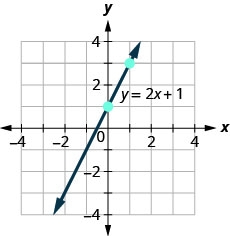Find the rise and run.Find the $$y$$-intercept of the line. The $$y$$-intercept is the point $$(0, 1)$$.The slope is the same as the coefficient of $$x$$ and the $$y$$-coordinate of the $$y$$-intercept is the same as the constant term.

##### Exercise $$\PageIndex{2}$$

Use the graph to find the slope and $$y$$-intercept of the line $$y=\frac{2}{3}x−1$$. Compare these values to the equation $$y=mx+b$$.slope $$m = \frac{2}{3}$$ and $$y$$-intercept $$(0,−1)$$

##### Exercise $$\PageIndex{3}$$

Use the graph to find the slope and $$y$$-intercept of the line $$y=\frac{1}{2}x+3$$. Compare these values to the equation $$y=mx+b$$.slope $$m = \frac{1}{2}$$ and $$y$$-intercept $$(0,3)$$

## Identify the Slope and $$y$$-Intercept From an Equation of a Line

In Understand Slope of a Line, we graphed a line using the slope and a point. When we are given an equation in slope–intercept form, we can use the $$y$$-intercept as the point, and then count out the slope from there. Let’s practice finding the values of the slope and $$y$$-intercept from the equation of a line.

##### Exercise $$\PageIndex{4}$$

Identify the slope and $$y$$-intercept of the line with equation $$y=−3x+5$$.

We compare our equation to the slope–intercept form of the equation.Write the equation of the line.Identify the slope.Identify the $$y$$-intercept.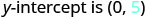##### Exercise $$\PageIndex{5}$$

Identify the slope and $$y$$-intercept of the line $$y=\frac{2}{5}x−1$$.

$$\frac{2}{5}$$; (0,−1)

##### Exercise $$\PageIndex{6}$$

Identify the slope and $$y$$-intercept of the line $$y=−\frac{4}{3}x+1$$.

$$-\frac{4}{3}$$; (0,1)

When an equation of a line is not given in slope–intercept form, our first step will be to solve the equation for $$y$$.

##### Exercise $$\PageIndex{7}$$

Identify the slope and $$y$$-intercept of the line with equation $$x+2y=6$$.

This equation is not in slope–intercept form. In order to compare it to the slope–intercept form we must first solve the equation for $$y$$.

 Solve for $$y$$. $$x+2y=6$$ Subtract x from each side.Divide both sides by $$2$$.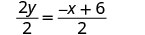Simplify.(Remember: $$\frac{a+b}{c} = \frac{a}{c} + \frac{b}{c}$$) Simplify.Write the slope–intercept form of the equation of the line.Write the equation of the line.Identify the slope.Identify the $$y$$-intercept.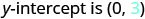##### Exercise $$\PageIndex{8}$$

Identify the slope and $$y$$-intercept of the line $$x+4y=8$$.

$$-\frac{1}{4}$$;(0,2)

##### Exercise $$\PageIndex{9}$$

Identify the slope and $$y$$-intercept of the line $$3x+2y=12$$.

$$-\frac{2}{3}$$;(0,6)

## Graph a Line Using its Slope and Intercept

Now that we know how to find the slope and $$y$$-intercept of a line from its equation, we can graph the line by plotting the $$y$$-intercept and then using the slope to find another point.

##### Exercise $$\PageIndex{10}$$: How to Graph a Line Using its Slope and Intercept

Graph the line of the equation $$y=4x−2$$ using its slope and $$y$$-intercept.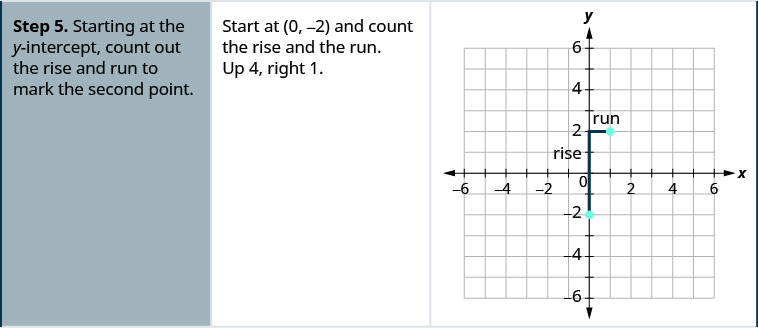##### Exercise $$\PageIndex{11}$$

Graph the line of the equation $$y=4x+1$$ using its slope and $$y$$-intercept.##### Exercise $$\PageIndex{12}$$

Graph the line of the equation $$y=2x−3$$ using its slope and $$y$$-intercept.##### GRAPH A LINE USING ITS SLOPE AND $$y$$-INTERCEPT.
1. Find the slope-intercept form of the equation of the line.
2. Identify the slope and $$y$$-intercept.
3. Plot the $$y$$-intercept.
4. Use the slope formula $$\frac{\text{rise}}{\text{run}}$$ to identify the rise and the run.
5. Starting at the $$y$$-intercept, count out the rise and run to mark the second point.
6. Connect the points with a line.
##### Exercise $$\PageIndex{13}$$

Graph the line of the equation $$y=−x+4$$ using its slope and $$y$$-intercept.

 $$y=mx+b$$ The equation is in slope–intercept form. $$y=−x+4$$ Identify the slope and $$y$$-intercept. $$m=−1$$ $$y$$-intercept is $$(0, 4)$$ Plot the $$y$$-intercept. See graph below. Identify the rise and the run. $$m = \frac{-1}{1}$$ Count out the rise and run to mark the second point. rise $$−1$$, run $$1$$ Draw the line.To check your work, you can find another point on the line and make sure it is a solution of the equation. In the graph we see the line goes through $$(4, 0)$$.
check.
$$\begin{array}{l}{y=-x+4} \\ {0\stackrel{?}{=}-4+4} \\ {0=0\checkmark}\end{array}$$
##### Exercise $$\PageIndex{14}$$

Graph the line of the equation $$y=−x−3$$ using its slope and $$y$$-intercept.##### Exercise $$\PageIndex{15}$$

Graph the line of the equation $$y=−x−1$$ using its slope and $$y$$-intercept.##### Exercise $$\PageIndex{16}$$

Graph the line of the equation $$y=−\frac{2}{3}x−3$$ using its slope and $$y$$-intercept.

 $$y=mx+b$$ The equation is in slope–intercept form. $$y=−\frac{2}{3}x−3$$ Identify the slope and $$y$$-intercept. $$m = -\frac{2}{3}$$; $$y$$-intercept is $$(0, −3)$$ Plot the $$y$$-intercept. See graph below. Identify the rise and the run. Count out the rise and run to mark the second point. Draw the line.##### Exercise $$\PageIndex{17}$$

Graph the line of the equation $$y=−\frac{5}{2}x+1$$ using its slope and $$y$$-intercept.##### Exercise $$\PageIndex{18}$$

Graph the line of the equation $$y=−\frac{3}{4}x−2$$ using its slope and $$y$$-intercept.##### Exercise $$\PageIndex{19}$$

Graph the line of the equation $$4x−3y=12$$ using its slope and $$y$$-intercept.

 $$4x−3y=12$$ Find the slope–intercept form of the equation. $$−3y=−4x+12$$ $$−\frac{3y}{3}=\frac{−4x+12}{−3}$$ The equation is now in slope–intercept form. $$y=\frac{4}{3}x−4$$ Identify the slope and $$y$$-intercept. $$m=\frac{4}{3}$$ $$y$$-ntercept is $$(0, −4)$$ Plot the $$y$$-intercept. See graph below. Identify the rise and the run; count out the rise and run to mark the second point. Draw the line.##### Exercise $$\PageIndex{20}$$

Graph the line of the equation $$2x−y=6$$ using its slope and $$y$$-intercept.##### Exercise $$\PageIndex{21}$$

Graph the line of the equation $$3x−2y=8$$ using its slope and $$y$$-intercept.We have used a grid with $$x$$ and $$y$$ both going from about $$−10$$ to $$10$$ for all the equations we’ve graphed so far. Not all linear equations can be graphed on this small grid. Often, especially in applications with real-world data, we’ll need to extend the axes to bigger positive or smaller negative numbers.

##### Exercise $$\PageIndex{22}$$

Graph the line of the equation $$y=0.2x+45$$ using its slope and $$y$$-intercept.

We’ll use a grid with the axes going from about $$−80$$ to $$80$$.

 $$y=mx+b$$ The equation is in slope–intercept form. $$y=0.2x+45$$ Identify the slope and $$y$$-intercept. $$m=0.2$$ The $$y$$-intercept is $$(0, 45)$$ Plot the $$y$$-intercept. See graph below. Count out the rise and run to mark the second point. The slope is $$m=0.2$$; in fraction form this means $$m=\frac{2}{10}$$. Given the scale of our graph, it would be easier to use the equivalent fraction $$m=\frac{10}{50}$$. Draw the line.##### Exercise $$\PageIndex{23}$$

Graph the line of the equation $$y=0.5x+25$$ using its slope and $$y$$-intercept.##### Exercise $$\PageIndex{24}$$

Graph the line of the equation $$y=0.1x−30$$ using its slope and $$y$$-intercept.Now that we have graphed lines by using the slope and $$y$$-intercept, let’s summarize all the methods we have used to graph lines. See Figure $$\PageIndex{2}$$.Figure $$\PageIndex{2}$$

## Choose the Most Convenient Method to Graph a Line

Now that we have seen several methods we can use to graph lines, how do we know which method to use for a given equation?

While we could plot points, use the slope–intercept form, or find the intercepts for any equation, if we recognize the most convenient way to graph a certain type of equation, our work will be easier. Generally, plotting points is not the most efficient way to graph a line. We saw better methods in sections 4.3, 4.4, and earlier in this section. Let’s look for some patterns to help determine the most convenient method to graph a line.

Here are six equations we graphed in this chapter, and the method we used to graph each of them.

$\begin{array}{lll}{\text{#1}}&{\text {Equation }} & {\text { Method }} \\ {\text{#2}}&{x=2} & {\text { Vertical line }} \\ {\text{#3}}&{y=4} & {\text { Hortical line }} \\ {\text{#4}}&{-x+2 y=6} & {\text { Intercepts }} \\ {\text{#5}}&{4 x-3 y=12} & {\text { Intercepts }} \\ {\text{#6}}&{y=4 x-2} & {\text { Slope-intercept }} \\{\text{#7}}& {y=-x+4} & {\text { Slope-intercept }}\end{array}$

Equations #1 and #2 each have just one variable. Remember, in equations of this form the value of that one variable is constant; it does not depend on the value of the other variable. Equations of this form have graphs that are vertical or horizontal lines.

In equations #3 and #4, both $$x$$ and $$y$$ are on the same side of the equation. These two equations are of the form $$Ax+By=C$$. We substituted $$y=0$$ to find the $$x$$-intercept and $$x=0$$ to find the $$y$$-intercept, and then found a third point by choosing another value for $$x$$ or $$y$$.

Equations #5 and #6 are written in slope–intercept form. After identifying the slope and $$y$$-intercept from the equation we used them to graph the line.

This leads to the following strategy.

##### STRATEGY FOR CHOOSING THE MOST CONVENIENT METHOD TO GRAPH A LINE

Consider the form of the equation.

• If it only has one variable, it is a vertical or horizontal line.
• $$x=a$$ is a vertical line passing through the $$x$$-axis at $$a$$.
• $$y=b$$ is a horizontal line passing through the $$y$$-axis at $$b$$.
• If $$y$$ is isolated on one side of the equation, in the form $$y=mx+b$$, graph by using the slope and $$y$$-intercept.
• Identify the slope and $$y$$-intercept and then graph.
• If the equation is of the form $$Ax+By=C$$, find the intercepts.
• Find the $$x$$- and $$y$$-intercepts, a third point, and then graph.
##### Exercise $$\PageIndex{25}$$

Determine the most convenient method to graph each line.

1. $$y=−6$$
2. $$5x−3y=15$$
3. $$x=7$$
4. $$y=\frac{2}{5}x−1$$.

1. $$y=−6$$
This equation has only one variable, $$y$$. Its graph is a horizontal line crossing the $$y$$-axis at $$−6$$.

2. $$5x−3y=15$$
This equation is of the form $$Ax+By=C$$. The easiest way to graph it will be to find the intercepts and one more point.

3. $$x=7$$
There is only one variable, $$x$$. The graph is a vertical line crossing the $$x$$-axis at $$7$$.

4. $$y=\frac{2}{5}x−1$$
Since this equation is in $$y=mx+b$$ form, it will be easiest to graph this line by using the slope and $$y$$-intercept.

##### Exercise $$\PageIndex{26}$$

Determine the most convenient method to graph each line:

1. $$3x+2y=12$$
2. $$y=4$$
3. $$y=\frac{1}{5}x−4$$
4. $$x=−7$$
1. intercepts
2. horizontal line
3. slope–intercept
4. vertical line
##### Exercise $$\PageIndex{27}$$

Determine the most convenient method to graph each line:

1. $$x=6$$
2. $$y=−\frac{3}{4}x+1$$
3. $$y=−8$$
4. $$4x−3y=−1$$
1. vertical line
2. slope–intercept
3. horizontal line
4. intercepts

## Graph and Interpret Applications of Slope–Intercept

Many real-world applications are modeled by linear equations. We will take a look at a few applications here so you can see how equations written in slope–intercept form relate to real-world situations.

Usually when a linear equation models a real-world situation, different letters are used for the variables, instead of $$x$$ and $$y$$. The variable names remind us of what quantities are being measured.

##### Exercise $$\PageIndex{28}$$

The equation $$F=\frac{9}{5}C+32$$ is used to convert temperatures, $$C$$, on the Celsius scale to temperatures, $$F$$, on the Fahrenheit scale.

1. Find the Fahrenheit temperature for a Celsius temperature of $$0$$.
2. Find the Fahrenheit temperature for a Celsius temperature of $$20$$.
3. Interpret the slope and $$F$$-intercept of the equation.
4. Graph the equation.

1. $$\begin{array}{ll}{\text { Find the Fahrenheit temperature for a Celsius temperature of } 0 .} & {F=\frac{9}{5} C+32} \\ {\text { Find } F \text { when } C=0 .} & {F=\frac{9}{5}(0)+32} \\ {\text { Simplify. }} & {F=32}\end{array}$$

2. \begin{array}{ll}{\text { Find the Fahrenheit temperature for a Celsius temperature of } 20 .} & {F=\frac{9}{5} C+32} \\ {\text { Find } F \text { when } C=20 .} & {F=\frac{9}{5}(20)+32} \\ {\text { Simplify. }} & {F=36+32} \\ {\text { Simplify. }} & {F=68}\end{array}

3. Interpret the slope and $$F$$-intercept of the equation.

Even though this equation uses $$F$$ and $$C$$, it is still in slope–intercept form.The slope, $$\frac{9}{5}$$, means that the temperature Fahrenheit ($$F$$) increases $$9$$ degrees when the temperature Celsius ($$C$$) increases $$5$$ degrees.

The $$F$$-intercept means that when the temperature is $$0°$$ on the Celsius scale, it is $$32°$$ on the Fahrenheit scale.

4. Graph the equation.

We’ll need to use a larger scale than our usual. Start at the $$F$$-intercept $$(0,32)$$ then count out the rise of $$9$$ and the run of $$5$$ to get a second point. See Figure $$\PageIndex{3}$$.Figure $$\PageIndex{3}$$
##### Exercise $$\PageIndex{29}$$

The equation $$h=2s+50$$ is used to estimate a woman’s height in inches, $$h$$, based on her shoe size, $$s$$.

1. Estimate the height of a child who wears women’s shoe size $$0$$.
2. Estimate the height of a woman with shoe size $$8$$.
3. Interpret the slope and $$h$$-intercept of the equation.
4. Graph the equation.
1. $$50$$ inches
2. $$66$$ inches
3. The slope, $$2$$, means that the height, $$h$$, increases by $$2$$ inches when the shoe size, $$s$$, increases by $$1$$. The $$h$$-intercept means that when the shoe size is $$0$$, the height is $$50$$ inches.##### Exercise $$\PageIndex{30}$$

The equation $$T=\frac{1}{4}n+40$$ is used to estimate the temperature in degrees Fahrenheit, $$T$$, based on the number of cricket chirps, $$n$$, in one minute.

1. Estimate the temperature when there are no chirps.
2. Estimate the temperature when the number of chirps in one minute is $$100$$.
3. Interpret the slope and $$T$$-intercept of the equation.
4. Graph the equation.
1. $$40$$ degrees
2. $$65$$ degrees
3. The slope, $$\frac{1}{4}$$, means that the temperature Fahrenheit ($$F$$) increases $$1$$ degree when the number of chirps, $$n$$, increases by $$4$$. The $$T$$-intercept means that when the number of chirps is $$0$$, the temperature is $$40°$$.The cost of running some types business has two components—a fixed cost and a variable cost. The fixed cost is always the same regardless of how many units are produced. This is the cost of rent, insurance, equipment, advertising, and other items that must be paid regularly. The variable cost depends on the number of units produced. It is for the material and labor needed to produce each item.

##### Exercise $$\PageIndex{31}$$

Stella has a home business selling gourmet pizzas. The equation $$C=4p+25$$ models the relation between her weekly cost, $$C$$, in dollars and the number of pizzas, $$p$$, that she sells.

1. Find Stella’s cost for a week when she sells no pizzas.
2. Find the cost for a week when she sells $$15$$ pizzas.
3. Interpret the slope and $$C$$-intercept of the equation.
4. Graph the equation.
 1. Find Stella's cost for a week when she sells no pizzas.Find $$C$$ when $$p=0$$.Simplify.Stella's fixed cost is $$25$$ when she sells no pizzas. 2. Find the cost for a week when she sells $$15$$ pizzas.Find $$C$$ when $$p=15$$.Simplify.Stella's costs are $$85$$ when she sells $$15$$ pizzas. 3. Interpret the slope and $$C$$-intercept of the equation.The slope, $$4$$, means that the cost increases by $$4$$ for each pizza Stella sells. The $$C$$-intercept means that even when Stella sells no pizzas, her costs for the week are $$25$$. 4. Graph the equation. We'll need to use a larger scale than our usual. Start at the $$C$$-intercept $$(0, 25)$$ then count out the rise of $$4$$ and the run of $$1$$ to get a second point.##### Exercise $$\PageIndex{32}$$

Sam drives a delivery van. The equation $$C=0.5m+60$$ models the relation between his weekly cost, $$C$$, in dollars and the number of miles, $$m$$, that he drives.

1. Find Sam’s cost for a week when he drives $$0$$ miles.
2. Find the cost for a week when he drives $$250$$ miles.
3. Interpret the slope and $$C$$-intercept of the equation.
4. Graph the equation.
1. $$60$$
2. $$185$$
3. The slope, $$0.5$$, means that the weekly cost, $$C$$, increases by $$0.50$$ when the number of miles driven, $$n$$, increases by $$1$$. The $$C$$-intercept means that when the number of miles driven is $$0$$, the weekly cost is $$60$$.##### Exercise $$\PageIndex{33}$$

Loreen has a calligraphy business. The equation $$C=1.8n+35$$ models the relation between her weekly cost, $$C$$, in dollars and the number of wedding invitations, $$n$$, that she writes.

1. Find Loreen’s cost for a week when she writes no invitations.
2. Find the cost for a week when she writes $$75$$ invitations.
3. Interpret the slope and $$C$$-intercept of the equation.
4. Graph the equation.
1. $$35$$
2. $$170$$
3. The slope, $$1.8$$, means that the weekly cost, $$C$$, increases by $$1.80$$ when the number of invitations, $$n$$, increases by $$1.80$$. The $$C$$-intercept means that when the number of invitations is $$0$$, the weekly cost is $$35$$.## Use Slopes to Identify Parallel Lines

The slope of a line indicates how steep the line is and whether it rises or falls as we read it from left to right. Two lines that have the same slope are called parallel lines. Parallel lines never intersect.Figure $$\PageIndex{4}$$.Figure $$\PageIndex{4}$$: Verify that both lines have the same slope, $$m=\frac{2}{5}$$, and different $$y$$-intercepts.

What about vertical lines? The slope of a vertical line is undefined, so vertical lines don’t fit in the definition above. We say that vertical lines that have different $$x$$-intercepts are parallel. See Figure $$\PageIndex{5}$$.Figure $$\PageIndex{5}$$: Vertical lines with different x-intercepts are parallel.
##### PARALLEL LINES

Parallel lines are lines in the same plane that do not intersect.

• Parallel lines have the same slope and different $$y$$-intercepts.
• If $$m_{1}$$ and $$m_{2}$$ are the slopes of two parallel lines then $$m_{1} = m_{2}$$.
• Parallel vertical lines have different $$x$$-intercepts.

Let’s graph the equations $$y=−2x+3$$ and $$2x+y=−1$$ on the same grid. The first equation is already in slope–intercept form: $$y=−2x+3$$. We solve the second equation for $$y$$:

\begin{aligned} 2x+y &=-1 \\ y &=-2x-1 \end{aligned}

Graph the lines.Notice the lines look parallel. What is the slope of each line? What is the $$y$$-intercept of each line?

$\begin{array}{lll} {y} & {=m x+b} & {y=m x+b} \\ {y} & {=-2 x+3} & {y=-2 x-1} \\ {m} & {=-2} & {m=-2}\\ {b} & {=3,(0,3)} & {b=-1,(0,-1)}\end{array}$

The slopes of the lines are the same and the $$y$$-intercept of each line is different. So we know these lines are parallel.

Since parallel lines have the same slope and different $$y$$-intercepts, we can now just look at the slope–intercept form of the equations of lines and decide if the lines are parallel.

##### Exercise $$\PageIndex{34}$$

Use slopes and $$y$$-intercepts to determine if the lines $$3x−2y=6$$ and $$y = \frac{3}{2}x + 1$$ are parallel.

$$\begin{array} {lrll} {\text { Solve the first equation for } y .} &{ 3 x-2 y} &{=} &{6}\\{} & {\frac{-2 y}{-2}} &{ =}&{-3 x+6 }\\ {} &{\frac{-2 y}{-2}}&{ =}&{\frac{-3 x+6}{-2}} \\ {} & {y }&{=}&{\frac{3}{2} x-3} \end{array}$$

The equation is now in slope–intercept form.

The equation of the second line is already in slope–intercept form.

Identify the slope and $$y$$-intercept of both lines.

$$\begin{array}{lll}{y=\frac{3}{2} x+1} & {} & {y=\frac{3}{2} x-3} \\ {y=m x+b} & {} & {y=m x+b}\\ {m=\frac{3}{2}} & {} & {m=\frac{3}{2}} \\ {y\text{-intercept is }(0, 1)} & {} & {y\text{-intercept is }(0, −3)} \end{array}$$

The lines have the same slope and different $$y$$-intercepts and so they are parallel. You may want to graph the lines to confirm whether they are parallel.

##### Exercise $$\PageIndex{35}$$

Use slopes and $$y$$-intercepts to determine if the lines $$2x+5y=5$$ and $$y=−\frac{2}{5}x−4$$ are parallel.

parallel

##### Exercise $$\PageIndex{36}$$

Use slopes and $$y$$-intercepts to determine if the lines $$4x−3y=6$$ and $$y=\frac{4}{3}x−1$$ are parallel.

parallel

##### Exercise $$\PageIndex{37}$$

Use slopes and $$y$$-intercepts to determine if the lines $$y=−4$$ and $$y=3$$ are parallel.

$$\begin{array}{llll}{\text{Write each equation in slope-intercept form.}} &{y=-4} & {\text { and }} &{ y=3} \\ {\text{Since there is no }x\text{ term we write }0x.} &{y=0 x-4} & {} &{y=0 x+3} \\ {\text{Identify the slope and }y\text{-intercept of both lines.}} &{y=m x+b} &{} & {y=m x+b} \\ {} &{m=0} &{} & {m=0} \\{} & {y\text {-intercept is }(0,4)} &{} & {y \text {-intercept is }(0,3)}\end{array}$$

The lines have the same slope and different $$y$$-intercepts and so they are parallel.

There is another way you can look at this example. If you recognize right away from the equations that these are horizontal lines, you know their slopes are both $$0$$. Since the horizontal lines cross the $$y$$-axis at $$y=−4$$ and at $$y=3$$, we know the $$y$$-intercepts are $$(0,−4)$$ and $$(0,3)$$. The lines have the same slope and different $$y$$-intercepts and so they are parallel.

##### Exercise $$\PageIndex{38}$$

Use slopes and $$y$$-intercepts to determine if the lines $$y=8$$ and $$y=−6$$ are parallel.

parallel

##### Exercise $$\PageIndex{39}$$

Use slopes and $$y$$-intercepts to determine if the lines $$y=1$$ and $$y=−5$$ are parallel.

parallel

##### Exercise $$\PageIndex{40}$$

Use slopes and $$y$$-intercepts to determine if the lines $$x=−2$$ and $$x=−5$$ are parallel.

$x=-2 \text { and } x=-5$

Since there is no $$y$$, the equations cannot be put in slope–intercept form. But we recognize them as equations of vertical lines. Their $$x$$-intercepts are $$−2$$ and $$−5$$. Since their $$x$$-intercepts are different, the vertical lines are parallel.

##### Exercise $$\PageIndex{41}$$

Use slopes and $$y$$-intercepts to determine if the lines $$x=1$$ and $$x=−5$$ are parallel.

parallel

##### Exercise $$\PageIndex{42}$$

Use slopes and $$y$$-intercepts to determine if the lines $$x=8$$ and $$x=−6$$ are parallel.

parallel

##### Exercise $$\PageIndex{43}$$

Use slopes and $$y$$-intercepts to determine if the lines $$y=2x−3$$ and $$−6x+3y=−9$$ are parallel. You may want to graph these lines, too, to see what they look like.

$$\begin{array} {llll} {\text { The first equation is already in slope-intercept form. }} & {y=2x-3}&{}&{} \\ \\ {\text { Solve the second equation for } y} & {-6x+3y} &{=}&{-9} \\{} & {3y}&{=}&{6x-9} \\ {}&{\frac{3y}{3} }&{=}&{\frac{6x-9}{3}} \\{} & {y}&{=}&{2x-3}\end{array}$$

The second equation is now in slope–intercept form as well.

Identify the slope and $$y$$-intercept of both lines.

$\begin{array}{lll}{y=2x-3} &{} & {y=2x-3} \\ {y=mx+b} &{} & {y=mx+b} \\ {m=2} &{} & {m=2} \\ {\text{The }y\text{-intercept is }(0 ,−3)} &{} & {\text{The }y\text{-intercept is }(0 ,−3)} \end{array} \nonumber$

The lines have the same slope, but they also have the same $$y$$-intercepts. Their equations represent the same line. They are not parallel; they are the same line.

##### Exercise $$\PageIndex{44}$$

Use slopes and $$y$$-intercepts to determine if the lines $$y=−\frac{1}{2}x−1$$ and $$x+2y=2$$ are parallel.

not parallel; same line

##### Exercise $$\PageIndex{45}$$

Use slopes and $$y$$-intercepts to determine if the lines $$y=\frac{3}{4}x−3$$ and $$3x−4y=12$$ are parallel.

not parallel; same line

## Use Slopes to Identify Perpendicular Lines

Let’s look at the lines whose equations are $$y=\frac{1}{4}x−1$$ and $$y=−4x+2$$, shown in Figure $$\PageIndex{5}$$.Figure $$\PageIndex{5}$$

These lines lie in the same plane and intersect in right angles. We call these lines perpendicular.

What do you notice about the slopes of these two lines? As we read from left to right, the line $$y=14x−1$$ rises, so its slope is positive. The line $$y=−4x+2$$ drops from left to right, so it has a negative slope. Does it make sense to you that the slopes of two perpendicular lines will have opposite signs?

If we look at the slope of the first line, $$m_{1}=14$$, and the slope of the second line, $$m_{2}=−4$$, we can see that they are negative reciprocals of each other. If we multiply them, their product is $$−1$$.

$\begin{array}{c}{m_{1} \cdot m_{2}} \\ {\frac{1}{4}(-4)} \\ {-1}\end{array}$

This is always true for perpendicular lines and leads us to this definition.

##### PERPENDICULAR LINES

Perpendicular lines are lines in the same plane that form a right angle.

If m1 and m2 are the slopes of two perpendicular lines, then:

$m_{1} \cdot m_{2}=-1 \text { and } m_{1}=\frac{-1}{m_{2}}$

Vertical lines and horizontal lines are always perpendicular to each other.

We were able to look at the slope–intercept form of linear equations and determine whether or not the lines were parallel. We can do the same thing for perpendicular lines.

We find the slope–intercept form of the equation, and then see if the slopes are negative reciprocals. If the product of the slopes is $$−1$$, the lines are perpendicular. Perpendicular lines may have the same $$y$$-intercepts.

##### Exercise $$\PageIndex{46}$$

Use slopes to determine if the lines, $$y=−5x−4$$ and $$x−5y=5$$ are perpendicular.

The first equation is already in slope–intercept form: $$\quad y=−5x−4$$

$$\begin{array} {llll} {\text{Solve the second equation for }y.} &{x-5y} &{=} &{5} \\{} &{-5 y} &{=} &{-x+5} \\ {} & {\frac{-5 y}{-5}} &{=} &{\frac{-x+5}{-5}} \\ {} &{y} &{=} &{\frac{1}{5} x-1} \end{array}$$

The second equation is now in slope-intercept form as well.

$$\begin{array} {lrllllll} {\text{Identify the slope of each line.}} &{y} &{=} &{-5 x-4} & {} &{y} &{=} &{\frac{1}{5} x-1} \\ {} &{y} &{=} &{m x+b} & {} &{y} &{=} &{m x+b}\\ {} &{m_{1}} &{=}&{-5} & {} &{m_{2}} &{=}&{\frac{1}{5}}\end{array}$$

The slopes are negative reciprocals of each other, so the lines are perpendicular. We check by multiplying the slopes,

$\begin{array}{l}{m_{1} \cdot m_{2}} \\ {-5\left(\frac{1}{5}\right)} \\ {-1\checkmark}\end{array}$

##### Exercise $$\PageIndex{47}$$

Use slopes to determine if the lines $$y=−3x+2$$ and $$x−3y=4$$ are perpendicular.

perpendicular

##### Exercise $$\PageIndex{48}$$

Use slopes to determine if the lines $$y=2x−5$$ and $$x+2y=−6$$ are perpendicular.

perpendicular

##### Exercise $$\PageIndex{49}$$

Use slopes to determine if the lines, $$7x+2y=3$$ and $$2x+7y=5$$ are perpendicular.

$$\begin{array}{lrlrl}{\text{Solve the equations for y.}} &{7 x+2 y} & {=3} & {2 x+7 y}&{=}&{5} \\{} & {2 y} & {=-7 x+3} & {7 y}&{=}&{-2 x+5} \\ {} &{\frac{2 y}{2}} & {=\frac{-7 x+3}{2} \quad} & {\frac{7 y}{7}}&{=}&{\frac{-2 x+5}{7}} \\ {} &{y} & {=-\frac{7}{2} x+\frac{3}{2}} &{y}&{=}&{\frac{-2}{7}x + \frac{5}{7}}\\ \\{\text{Identify the slope of each line.}} & {y}&{=m x+b} &{y}&{=}&{m x+b} \\{} & {m_{1}} & {=-\frac{7}{2} }&{ m_{2}}&{=}&{-\frac{2}{7}}\end{array}$$

The slopes are reciprocals of each other, but they have the same sign. Since they are not negative reciprocals, the lines are not perpendicular.

##### Exercise $$\PageIndex{50}$$

Use slopes to determine if the lines $$5x+4y=1$$ and $$4x+5y=3$$ are perpendicular.

not perpendicular

##### Exercise $$\PageIndex{51}$$

Use slopes to determine if the lines $$2x−9y=3$$ and $$9x−2y=1$$ are perpendicular.

not perpendicular

##### Note

Access this online resource for additional instruction and practice with graphs.

## Key Concepts

• The slope–intercept form of an equation of a line with slope mm and $$y$$-intercept, $$(0,b)$$ is, $$y=mx+b$$.
• Graph a Line Using its Slope and $$y$$-Intercept
1. Find the slope-intercept form of the equation of the line.
2. Identify the slope and $$y$$-intercept.
3. Plot the $$y$$-intercept.
4. Use the slope formula $$m = \dfrac{\text{rise}}{\text{run}}$$ to identify the rise and the run.
5. Starting at the $$y$$-intercept, count out the rise and run to mark the second point.
6. Connect the points with a line.
• Strategy for Choosing the Most Convenient Method to Graph a Line: Consider the form of the equation.
• If it only has one variable, it is a vertical or horizontal line.
$$x = a$$ is a vertical line passing through the $$x$$-axis at a.
$$y = b$$ is a horizontal line passing through the $$y$$-axis at $$b$$.
• If $$y$$ is isolated on one side of the equation, in the form $$y=mx+b$$, graph by using the slope and $$y$$-intercept.
Identify the slope and $$y$$-intercept and then graph.
• If the equation is of the form $$Ax+By=C$$, find the intercepts.
Find the $$x$$- and $$y$$-intercepts, a third point, and then graph.
• Parallel lines are lines in the same plane that do not intersect.
• Parallel lines have the same slope and different $$y$$-intercepts.
• If $$m_1$$ and $$m_2$$ are the slopes of two parallel lines then $$m_1 = m_2$$.
• Parallel vertical lines have different $$x$$-intercepts.
• Perpendicular lines are lines in the same plane that form a right angle.
• If $$m_1$$ and $$m_2$$ are the slopes of two perpendicular lines, then $$m_1\cdot m_2=−1$$ and $$m_1=\frac{−1}{m_2}$$.
• Vertical lines and horizontal lines are always perpendicular to each other.

## Glossary

parallel lines
Lines in the same plane that do not intersect.
perpendicular lines
Lines in the same plane that form a right angle.
slope-intercept form of an equation of a line
The slope–intercept form of an equation of a line with slope mm and $$y$$-intercept, $$(0,b)$$ is, $$y=mx+b$$.

4.5: Use the Slope–Intercept Form of an Equation of a Line is shared under a CC BY-NC-SA license and was authored, remixed, and/or curated by OpenStax.